# Malgrange-Ehrenpreis theorem

Briefly said: Every constant-coefficients linear partial differential equation oncan be solved.

Letbe a polynomial invariables,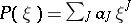, where the sum is a finite one, eachis a multi-index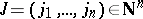, and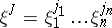. Toone associates a constant-coefficient linear partial differential operator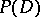, obtained by replacing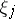by().

Although several special cases had been known for a long time, it is only in the mid 1950s that it has been shown that for every non-zero constant-coefficient linear partial differential operator the equation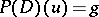can be solved for all functions or distributions (cf. also Generalized function)on. In particular, there is a distributionsuch that, whereis the Dirac mass at(cf. also Dirac delta-function). Such anis called a fundamental solution. This is the celebrated Malgrange–Ehrenpreis theorem (established independently by B. Malgrange [a8] and L. Ehrenpreis [a1]). In case of the Laplace operator, the Newton potential (up to some factor) is a fundamental solution.

Ifis any distribution onwith compact support, by using a fundamental solutionit is immediate to solve the equation. Indeed (denoting convolution, cf. also Convolution of functions),The Malgrange–Ehrenpreis theory goes further. One can solve the equation for non-compactly supported right-hand sideby exhaustingby balls, and by solving the equation on each ball. The process is made to converge by corrections based on the approximation of local solutions to the homogeneous equation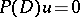by global solutions. The space of solutions to a linear homogeneous ordinary differential equation, with constant coefficients (for example,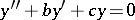), is spanned by exponential solutions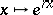(in the example,is a root of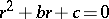), or by exponential and possibly polynomial-exponential solutions (in the case of a double root). Similarly, the solutions tocan be approximated, on convex sets, by linear combinations of polynomial-exponential solutions, functions of the type, whereis a polynomial and necessarily. See [a2], [a8], [a5], (7.3.6). More generally, convolution equations can be considered.

Most often, the Malgrange–Ehrenpreis theorem is attacked by using the Fourier transform. Ifis a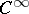-function on, say with compact support, its Fourier transform is defined byand one has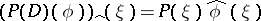. Looking for a fundamental solution basically consists in solving the equation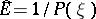. This is of course totally elementary in case the function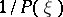is integrable on, since then, by inverse Fourier transformation, one can define (classically)by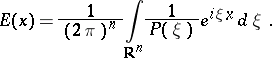(For example, withdenoting the usual Laplacian, the operator, for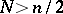, for which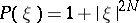.)

In general, one has to face two problems: the lack of integrability at infinity of, and the real zeros of the polynomial. Roughly speaking, since the two problems are not disjoint, one overcomes the first difficulty by the calculus of distributions, and the second one by switching to the complex domain. Both Malgrange and Ehrenpreis used the Hahn–Banach theorem. By now (1998) things have been much simplified, and a quick "constructive" proof of the Malgrange–Ehrenpreis theorem can be found in [a5], (7.3.10), see also [a3], (1.56). A formula claimed to be more explicit was recently given by H. König [a7].

The solvability of constant-coefficients linear partial differential operators can also be studied with pure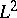methods. There is a remarkable fundamental result by L.V. Hörmander [a4], (2.6), whose proof is totally elementary, brief, and a masterpiece:

Ifis a bounded set in, and ifis non-zero, there exists a constant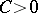such that for every-functionwith compact support in,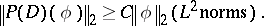It then follows, from elementary Hilbert space theory, that the adjoint operator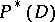(associated to the polynomial) is solvable in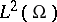. From there, one can recover the Malgrange–Ehrenpreis theorem. See [a10], or [a9] for an elementary exposition. However, the problem being not only to show the existence of a fundamental solution, but to get solutions with good properties, the construction in [a5], Chap. 7, seems close to being optimal, see [a6], Chap. 10.

The situation is radically different for operators with variable coefficients (see Lewy operator and Mizohata operator).